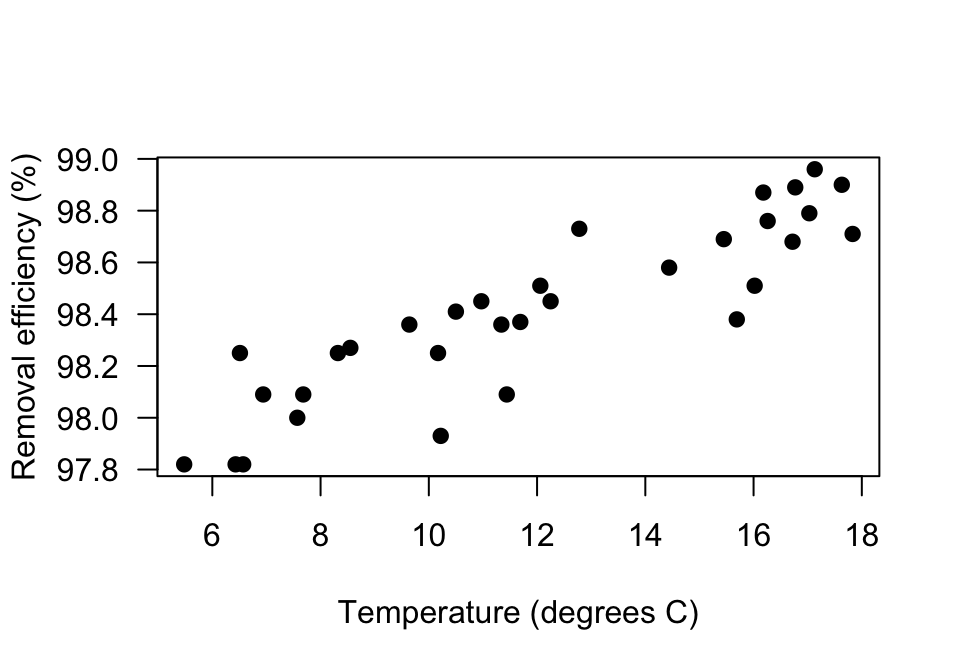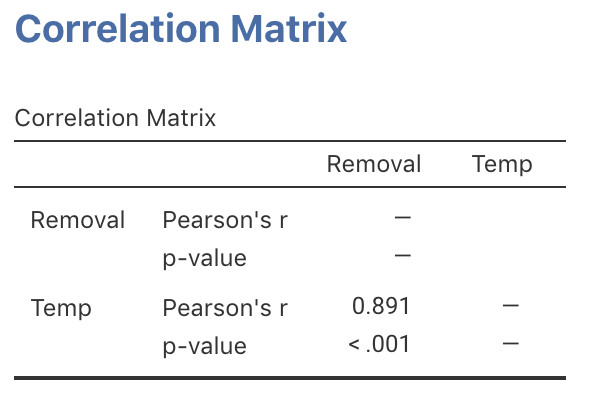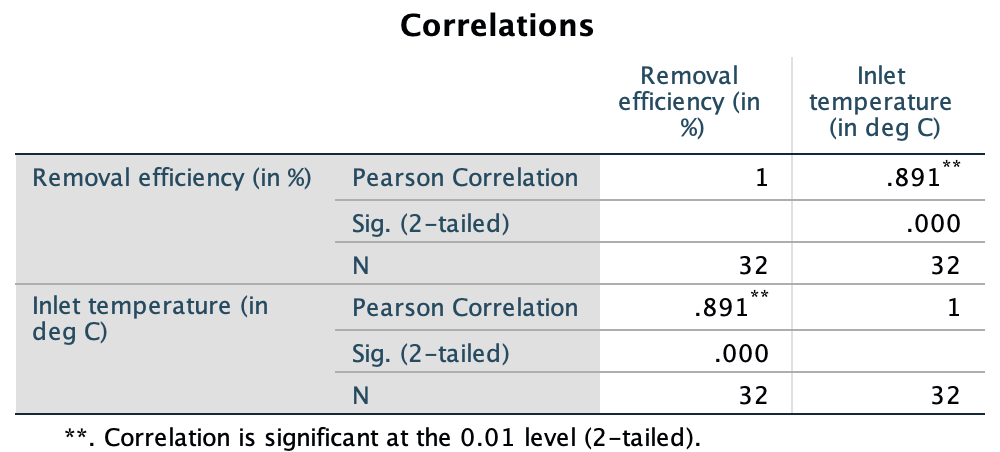## 34.5 Example: Removal efficiencyIn wastewater treatment facilities, air from biofiltration is passed through a membrane and dissolved in water, and is transformed into harmless byproducts. The removal efficiency $$y$$ (in %) may depend on the inlet temperature (in $$^\circ$$C; $$x$$).

The RQ is

In treating biofiltation wastewater, is the removal efficiency associated with the inlet temperature?

The population parameter is $$\rho$$, the correlation between the removal efficiency and inlet temperature.

A scatterplot of $$n=32$$ samples (Fig. 34.9) suggests an approximately linear relationship . The output (jamovi: Fig. 34.10; SPSS: Fig. 34.11) shows that the sample correlation coefficient is $$r=0.891$$, and so $$R^2 = (0.891)^2 = 79.4$$%. This means that about 79.4% of the variation in removal efficiency can be explained by knowing the inlet temperature.FIGURE 34.9: The relationship between removal efficiency and inlet temperature

To test if a relationship exists in the population, write:

• $$H_0$$: $$\rho = 0$$;
• $$H_1$$: $$\rho \ne 0$$: Two-tailed (as implied by the RQ).

The software output (jamovi: Fig. 34.10; SPSS: Fig. 34.11) shows that $$P < 0.001$$ (which is what $$P = 0.000$$ in SPSS means). We conclude:

The sample presents very strong evidence (two-tailed $$P < 0.001$$) that removal efficiency depends on the inlet temperature ($$r = 0.891$$; $$n = 32$$) in the population.

The relationship is approximately linear and there is no obvious non-constant variance, and the sample size is larger than 25, so the hypothesis test results will be statistically valid.FIGURE 34.10: jamovi output for the removal-efficiency dataFIGURE 34.11: SPSS output for the removal-efficiency data

### References

Chitwood DE, Devinny JS. Treatment of mixed hydrogen sulfide and organic vapors in a rock medium biofilter. Water Environment Research. Water Environment Federation; 2001;73(4):426–35.
Devore JL, Berk KN. Modern mathematical statistics with applications. Thomson Higher Education; 2007.